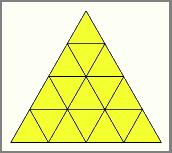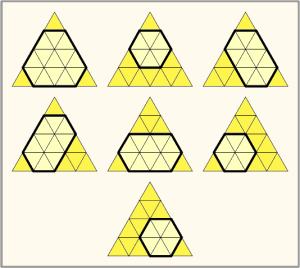Problem Statement

Petya likes triangles. He draws a trianglar grid using the following process: He starts off with an equilateral triangle. He then draws points on the edges of this triangle, dividing each edge up into N equal-length segments. Next, he connects each pair of points that is at equal distance from some vertex of the triangle with a straight line, ending up with N*N smaller equilateral triangles. An example of this figure with N = 4 is shown below.However, he likes hexagons more than he likes triangles. How many non-degenerate convex hexagons can be formed using the line segments in his figure? This number can be very big, so return it modulo 1000000007.

Definition

 Class: ConvexHexagons Method: find Parameters: int Returns: int Method signature: int find(int N) (be sure your method is public)

Notes

-The length of each edge of every hexagon must be greater than zero.

Constraints

-N will be between 1 and 500000, inclusive.

Examples

0)

 4
Returns: 7
 There are 7 hexagons:1)

 7
Returns: 232
2)

 104
Returns: 635471838
3)

 1
Returns: 0

Problem url:

http://www.topcoder.com/stat?c=problem_statement&pm=10715

Problem stats url:

http://www.topcoder.com/tc?module=ProblemDetail&rd=14179&pm=10715

Seyaua

Testers:

Rustyoldman , timmac , StevieT , maksay , K.A.D.R , Seyaua

Problem categories:

Dynamic Programming, Math